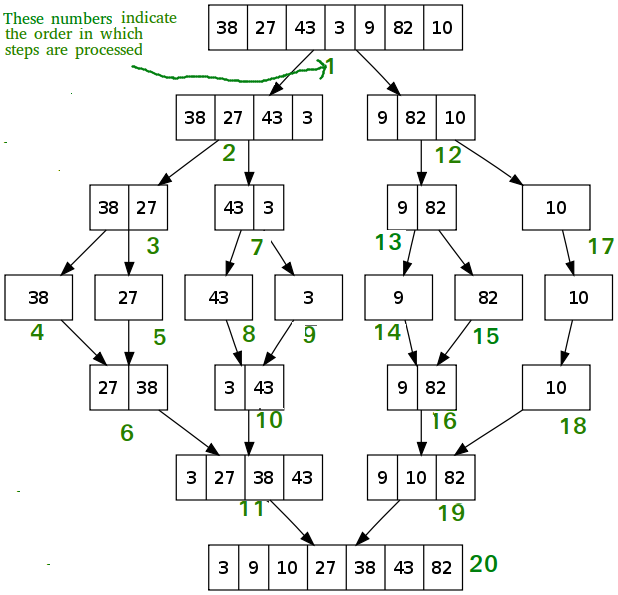# Divide and Conquer

Some Quizzes on Divide and Conquer

## What is Divide and Conquer?

Divide and Conquer is an algorithmic paradigm in which the problem is solved using the Divide, Conquer, and Combine strategy.

A typical Divide and Conquer algorithm solves a problem using following three steps:

1. Divide: This involves dividing the problem into smaller sub-problems.
2. Conquer: Solve sub-problems by calling recursively until solved.
3. Combine: Combine the sub-problems to get the final solution of the whole problem.

## Standard algorithms that follow Divide and Conquer algorithm

The following are some standard algorithms that follow Divide and Conquer algorithm.

1. Quicksort is a sorting algorithm. The algorithm picks a pivot element and rearranges the array elements so that all elements smaller than the picked pivot element move to the left side of the pivot, and all greater elements move to the right side. Finally, the algorithm recursively sorts the subarrays on the left and right of the pivot element.
2. Merge Sort is also a sorting algorithm. The algorithm divides the array into two halves, recursively sorts them, and finally merges the two sorted halves.
3. Closest Pair of Points The problem is to find the closest pair of points in a set of points in the x-y plane. The problem can be solved in O(n^2) time by calculating the distances of every pair of points and comparing the distances to find the minimum. The Divide and Conquer algorithm solves the problem in O(N log N) time.
4. Strassen’s Algorithm is an efficient algorithm to multiply two matrices. A simple method to multiply two matrices needs 3 nested loops and is O(n^3). Strassen’s algorithm multiplies two matrices in O(n^2.8974) time.
5. Cooley–Tukey Fast Fourier Transform (FFT) algorithm is the most common algorithm for FFT. It is a divide and conquer algorithm which works in O(N log N) time.
6. Karatsuba algorithm for fast multiplication does the multiplication of two n-digit numbers in at mostsingle-digit multiplications in general (and exactlywhen n is a power of 2). It is, therefore, faster than the classical algorithm, which requires n2 single-digit products. If n = 210 = 1024, in particular, the exact counts are 310 = 59, 049 and (210)2 = 1, 048, 576, respectively.

## Example of Divide and Conquer algorithm

A classic example of Divide and Conquer is Merge Sort demonstrated below. In Merge Sort, we divide array into two halves, sort the two halves recursively, and then merge the sorted halves.Topics:

Introduction:

Some Standard algorithms:

Binary Search based problems:

Some other Practic problems: